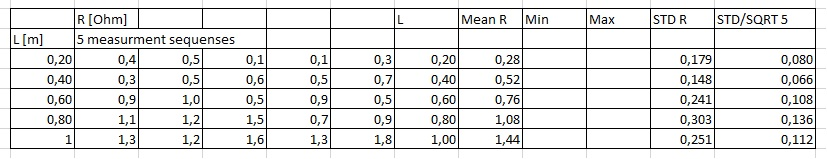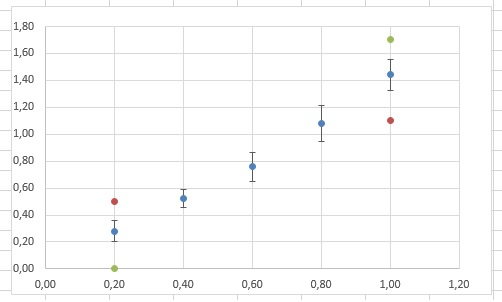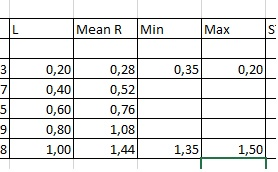# Data analysis 1, linear relations,not a proportionality

Up a level : IB Physics
Previous page : Data analysis 1, linear relations, proportionalities
Next page : Data analysis 1, linear relations, proportionalities, Zooming inData Analysis 1, linear relations, non proportionalities

One has to be really careful when assuming that one has proportionality, even in cases where one would expect a proportionality. So let us analyse the same data as in the previous page, (you can find it there) but now we will analyse it, not assuming that we have a proportionality, but still that we have a linear relation. We will thus get a relation in the form

R=(m±uncertainty in m)L+c±uncertainty in c

You can do this analysis using the leaf  “The Data, part 1, II”. We start as before to get the following table:Next we make a scatter plot, including the error bars, as on the previous page.Next we will add values to the Min and Max columns. This to enable us to get two lines at the minimum and maximum slope that would fit our data. The actual values you will use are not that important since you will change them anyhow.As in the previous page we can now right click somewhere on the empty part of the graph to be able to add some data. We add a series using L as our x-values and Min as our y-values, and then one series with L as our x-values and Max as our y-values. A graph of this should look something like this:Next we right click on one of the new points, say the green upper one, and then we add a trend line, including the equation. Next we do the same with the red points. We should now have something like this.Then we change the values in the Min- and Max-column to make our lines fit the data. This is what I got.You should try to make the lines fit somewhat trough the error bars, and the lines should (usually) cross somewhere in the middle of the graph. In this case one could suspect a non linear relation (why?), so one could possibly do more measurements, but let us continue assuming it is linear.  The average slope is  1.4375 with an uncertainty of  (max–min)/2=0.1875. If we round 1.4375 to 1.4 we will add an error of 0.0373 to get an error of 0.225 that we round to 0.3. This gives us 1.4±0.3.

Next we do the same with the y-intercept, or rather the R-intercept. We get an average of -0.0125 with an uncertainty of 0.1125. We round this to 0±0.2, or simply ±0.2. This will finally give us

R=(1.4±0.3)L±0.2

This works well with the previous result R=(1.4±0.2)L, and consistent with the idea that it could  be a proportionality.

The reason we got I slightly larger uncertainty is that we don’t assume the fisrst point is known exactly.Up a level : IB Physics
Previous page : Data analysis 1, linear relations, proportionalities
Next page : Data analysis 1, linear relations, proportionalities, Zooming inLast modified: May 1, 2018 @ 21:11